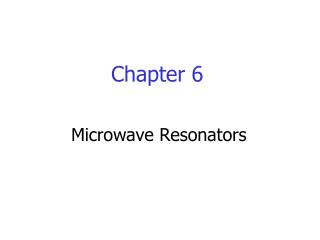# Chapter 6 - PowerPoint PPT PresentationDownload PresentationChapter 6

Chapter 6Download Presentation## Chapter 6

- - - - - - - - - - - - - - - - - - - - - - - - - - - E N D - - - - - - - - - - - - - - - - - - - - - - - - - - -
##### Presentation Transcript

1. Chapter 6 Microwave Resonators

2. Microwave resonators are used in a variety of applications, including filters, oscillators, frequency meters, and tuned amplifiers. • The operation of microwave resonators is very similar to that of the lumped-element resonators (such as parallel and series RLC resonant circuits) of circuit theory. RLC Series Resonant Circuit Vout Vin

3. RLC Parallel Resonant Circuit

4. Lossy resonators • Lossy resonator can be modeled as a lossless resonator (R=0) whose resonant frequency has been replaced with a complex effective resonant frequency. • For series resonators: • For parallel resonators:

5. Loaded and Unloaded Q • Unloaded Q: only account for the characteristic of the resonant circuit itself. • Loaded QL: account for Q value including the resonant circuit and the loading effect. It will be lower than unload Q.

6. Transmission Line Resonators • Short-Circuited /2 Line:

7. b a Example 6.1 : A /2 coaxial resonator with b=0.25inch, a=0.075inch, r=2.08 (Teflon), tan=0.01, c =5.8107 (copper). If f0=1GHz, find l, Q, and RLC equivalent circuit? Solution: l=/2

8. b a Example 6.2 : A /2 coaxial resonator with b=4mm, a=1mm, If f0=5GHz, compare the Q of an air-filled coaxial line resonator to that of a Teflon-filled coaxial line resonator? l=/2 Solution:

9. Short-Circuited /4 Line:

10. Open-Circuited /2 Line:

11. Open-Circuited /4 Line:

12. Example 6.3 : Design an open-circuit /2 microstrip line resonator with Z0=50. The substrate is FR4 ( r=4.5, h=1.6mm, tan=0.02, c =5.8107). If f0=5GHz, find l, W, Q, and RLC equivalent circuit? Solution:

13. Example 6.4 : Consider a /2 resonator formed by Z0=50 open-circuited microstrip line. The substrate is Teflon (r=2.08, tan=0.0004) with a thickness (h) of 0.159cm. If f0=5GHz, Compute the length of line and the Q of resonator? Ignore fringing fields at the end of the line. Solution: